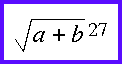## Can your browser display Presentation MathML?

Below you should see an equation inlined within some text

some text, some text, ${\left[a+b\right]}^{260}+{\left\{a+b\right\}}_{i}$ some text.

This picture shows a possible rendering of it:Below is an equation with a radical:

${\sqrt{a+b}}^{27}$

a rendering of which is shown below:If your browser displays those equations correctly, it is enabled to display Presentation MathML.